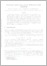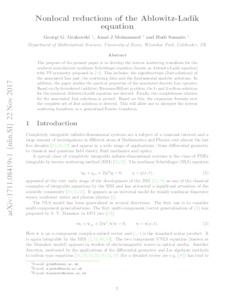# Nonlocal Reductions of the Ablowitz–Ladik Equation

Grahovski, GG and Mohammed, AJ and Susanto, H (2018) 'Nonlocal Reductions of the Ablowitz–Ladik Equation.' Theoretical and Mathematical Physics, 197 (1). 1412 - 1429. ISSN 0040-5779Preview
Text
1711.08419v1.pdf - Accepted Version

Download (429kB) | Preview

## Abstract

Our purpose is to develop the inverse scattering transform for the nonlocal semidiscrete nonlinear Schrödinger equation (called the Ablowitz–Ladik equation) with PT symmetry. This includes the eigenfunctions (Jost solutions) of the associated Lax pair, the scattering data, and the fundamental analytic solutions. In addition, we study the spectral properties of the associated discrete Lax operator. Based on the formulated (additive) Riemann–Hilbert problem, we derive the one- and two-soliton solutions for the nonlocal Ablowitz–Ladik equation. Finally, we prove the completeness relation for the associated Jost solutions. Based on this, we derive the expansion formula over the complete set of Jost solutions. This allows interpreting the inverse scattering transform as a generalized Fourier transform.

Item Type: Article integrable system, soliton, PT symmetry, nonlocal reduction, Riemann–Hilbert problem Q Science > QA Mathematics Faculty of Science and Health > Mathematical Sciences, Department of Elements 29 Nov 2018 15:47 29 Nov 2018 16:15 http://repository.essex.ac.uk/id/eprint/21352

### Actions (login required)View Item